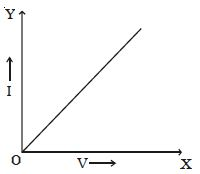# Describe Ohm’s Law with Equation

George Simon Ohm established the relationship between potential difference and current, which is known as Ohm’s law. The current flowing through a conductor is,

I = nAevd

But vd = eE/m . τ

so, I = nAe (eE/m) . τ

Then, I = (nAe2/ mL) . τV

Where, V is the potential difference. The quantity [mL/(nAe2 τ)] is a constant for a given conductor, called electrical resistance (R).

so, I α V

The law states that, at a constant temperature, the steady current flowing through a conductor is directly proportional to the potential difference between the two ends of the conductor.

(i.e) I α V or, I = (1/R).V

V = IR or R = V/I

Resistance of a conductor is defined as the ratio of potential difference across the conductor to the current flowing through it. The unit of resistance is ohm (Ω) The reciprocal of resistance is conductance. Its unit is mho (Ω-1).Fig: V−I graph of an ohmic conductor.

Since, potential difference V is proportional to the current I, the graph (Figure) between V and I is a straight line for a conductor. Ohm’s law holds good only when a steady current flows through a conductor.